# Mysteries - Who invented the harmonic mean and what is its purpose and why does it work?

• This post is deleted!

• [Originally posted in the Discussions]

Module 1 Week 4 Day 15 Challenge Explanation Part 4

Why doesn't the simple arithmetic mean work here? Who invented the harmonic mean and what is its purpose and why does it work?

And why can't I find the live chat on youtube anymore? I don't get the questions and stuff in the live chat and I would like to see it again.

• [Response credit @thomas]

This is a great question! I can't say much about the live chat problem, but I can explain why the harmonic mean is there and why it's helpful.

There are actually lots of different "means", and they each serve a different purpose. The "original" arithmetic mean, $$\frac{a + b}{ 2},$$ is useful for finding the number that's exactly between two other numbers.

So why are there other means? There is a quadratic mean, $$\frac{\sqrt{(a^2+b^2}}{ 2},$$ a geometric mean, $$\sqrt{a \times b},$$ and there is a harmonic mean, $$\frac{2}{\frac{1}{a} + \frac{1}{b}}.$$ These different types of means exist when you need to calculate a "middle" value in a weird situation, where things aren't related to each other in a "normal" or "proportional" way.

$$\text{ quadratic mean:} \frac{\sqrt{(a^2+b^2}}{ 2}$$
$$\text{ geometric mean:} \sqrt{a \times b}$$
$$\text{ harmonic mean:} \frac{2}{\frac{1}{a} + \frac{1}{b}}.$$

For example, let's say a rabbit population is doubling every second. At $$t = 4$$ seconds, there are $$16$$ rabbits. At $$t = 6$$ seconds, there are $$64$$ rabbits. How many rabbits are there at $$t = 5$$ seconds?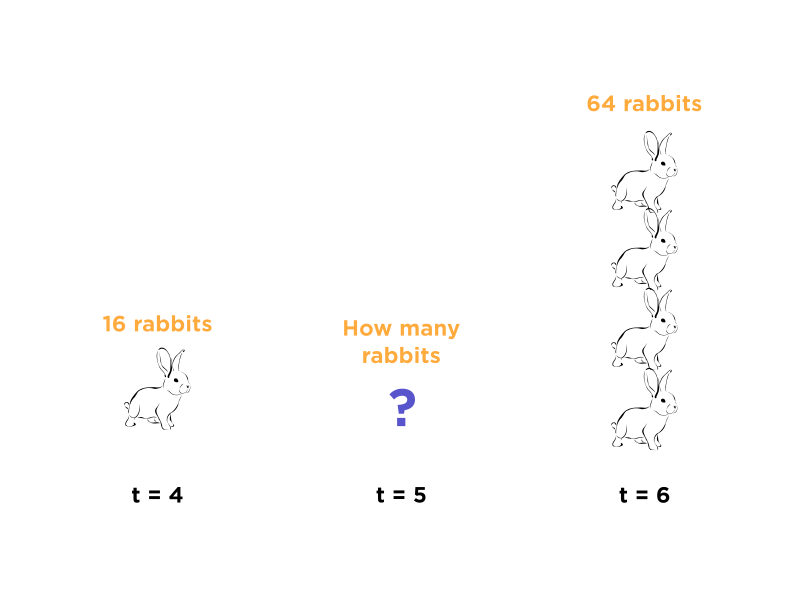Well, $$5$$ seconds is right in the middle between $$4$$ and $$6$$ seconds. So, is it just the arithmetic mean, $$\frac{ 16 + 64}{2} = 40?$$ You can probably imagine that it's not! If you see the pattern, you can guess that there should be $$32$$ rabbits after $$5$$ seconds. So what's the relation? It's actually the geometric mean! If you do the math, $$\sqrt{16 \times 64} = 32.$$ So, this is a "weird" situation in which things are related to each other in a "geometric" or "exponential" way, and not a "linear" way.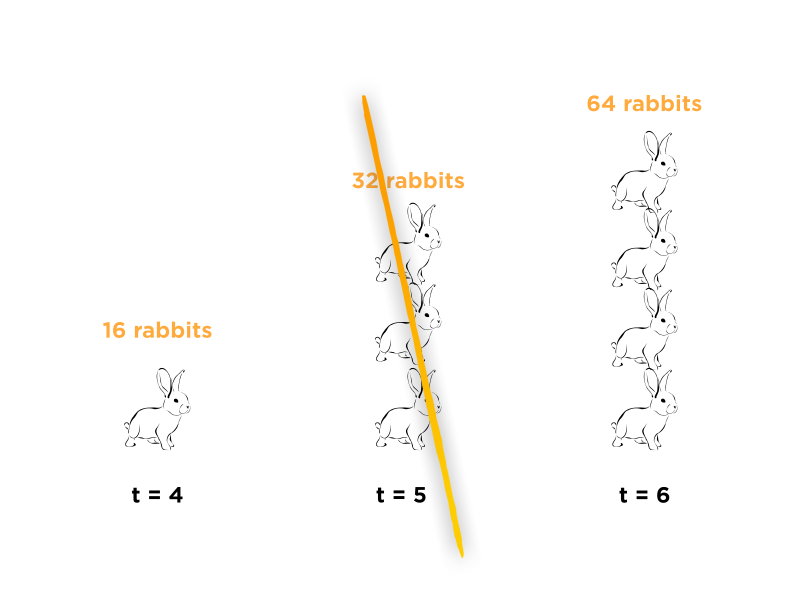In the challenge problem, you're given a problem about rates. You travel some distance at $$4 \frac{\text{mi}}{\text{hr}},$$ then travel over the same distance at $$12 \frac{\text{mi}}{\text{hr}}.$$ What's the average or "mean" speed? Isn't it just $$8 \frac{\text{mi}}{\text{hr}}?$$ Not quite!

Why are things "weird" here? Let's think of it like this: If I travelled at $$8 \frac{\text{mi}}{\text{hr}}$$ for an hour, then travelled at $$12 \frac{\text{mi}}{\text{hr}}$$, I'd definitely agree that the average speed is $$10 \frac{\text{mi}}{\text{hr}}.$$ Things are pretty normal here: If I want to calculate the total distance traveled, it's just $$8 + 12 = 20.$$ Then, if I want to calculate the total time traveled, it's just $$1 + 1 = 2.$$ So the average or overall speed is just
$$\frac{20 \text{mi}}{2 \text{hr}} = 10 \frac{\text{mi}}{\text{hr}}.$$ It works!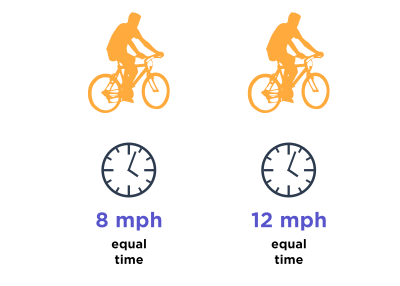But in that case, it only worked so well because for both the $$8 \frac{\text{mi}}{\text{hr}}$$ part and the $$12 \frac{\text{mi}}{\text{hr}}$$, I traveled for the same amount of time. In the challenge problem, I travel at $$8 \frac{\text{mi}}{\text{hr}}$$ and $$12 \frac{\text{mi}}{\text{hr}}$$ for the same amount of distance. In fact, I'm going to spend less time travelling at $$12 \frac{\text{mi}}{\text{hr}}$$ than $$8 \frac{\text{mi}}{\text{hr}}$$ (can you see why?). So I shouldn't "count" the $$12 \frac{\text{mi}}{\text{hr}}$$ as "much". Because of this, the "average" speed should really be closer to $$8$$ then $$12!$$ That's why doing just $$\frac{8 + 12}{2}$$ wouldn't make too much sense.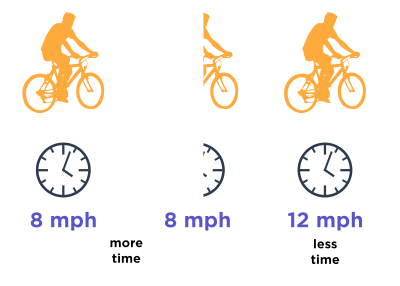So... now why would the harmonic mean make more sense? Professor Loh gave a good algebraic explanation, but let's see if there is a good reason to think that the average speed would even look like that.

What is a harmonic mean? It looks like $$\frac{1}{\frac{\frac{1}{x} + \frac{1}{y}}{2}}.$$ In other words, you flip both the $$x$$ and $$y,$$ take the average, then flip it again. Why do we have to flip so many times for this problem? Why are things so "flipped"?

## Why are things so "flipped"?

Let's say I run three times as fast as my friend (so our speeds are $$3:1$$).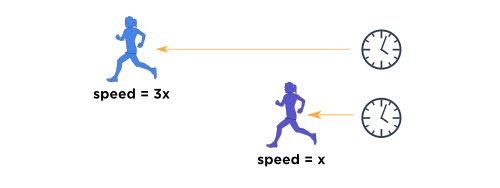Let's both run $$1$$ mile. What is the ratio between our finishing times? Since I'm twice as fast, I should take one-third as much time! So, our times traveled are actually in the ratio $$1:3.$$ It's flipped!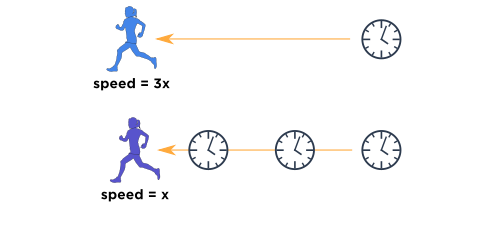Similarly, in the challenge problem, since the speeds up and down are in the ratio $$4:12,$$ and the distances are the same, the times traveled should be in the "flipped" ratio $$12:4.$$ That's why it's reasonable to see that to get the final answer, a lot of "flipping" is involved!

If you want to see where else the harmonic mean might show up, try these problems. One is "normal", but the other is "weird", so careful! It might not exactly be the harmonic mean, but it will look very similar.

If I can build $$2$$ houses per hour, and my friend can build 3 houses per hour, how many houses can we build per hour if we work together? (Hint: Is it just $$2 + 3?$$ Why does that make sense?)

If it takes me $$2$$ hours to build a house, and it takes my friend $$3$$ hours to build a house, how many hours will it take for us to build a house if we work together? (Hint: Why isn't this normal? Can you restate this problem a bit differently so it looks like the previous problem? Try to see why this problem needs a lot of "flipping"!)

Good luck, and happy learning!

Thomas
The Daily Challenge Team

0

• @debbie

Similarly, in the challenge problem, since the speeds up and down are in the ratio 4:12, and the distances are the same, the times traveled should be in the "flipped" ratio 12:4. That's why it's reasonable to see that to get the final answer, a lot of "flipping" is involved!

Why do we flip again at the end, then?